Test: Filter Coefficients Quantization

# Test: Filter Coefficients Quantization

Test Description

## 10 Questions MCQ Test Signals and Systems | Test: Filter Coefficients Quantization

Test: Filter Coefficients Quantization for Electrical Engineering (EE) 2023 is part of Signals and Systems preparation. The Test: Filter Coefficients Quantization questions and answers have been prepared according to the Electrical Engineering (EE) exam syllabus.The Test: Filter Coefficients Quantization MCQs are made for Electrical Engineering (EE) 2023 Exam. Find important definitions, questions, notes, meanings, examples, exercises, MCQs and online tests for Test: Filter Coefficients Quantization below.
Solutions of Test: Filter Coefficients Quantization questions in English are available as part of our Signals and Systems for Electrical Engineering (EE) & Test: Filter Coefficients Quantization solutions in Hindi for Signals and Systems course. Download more important topics, notes, lectures and mock test series for Electrical Engineering (EE) Exam by signing up for free. Attempt Test: Filter Coefficients Quantization | 10 questions in 10 minutes | Mock test for Electrical Engineering (EE) preparation | Free important questions MCQ to study Signals and Systems for Electrical Engineering (EE) Exam | Download free PDF with solutions
 1 Crore+ students have signed up on EduRev. Have you?
Test: Filter Coefficients Quantization - Question 1

### The system function of a general IIR filter is given as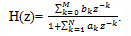Detailed Solution for Test: Filter Coefficients Quantization - Question 1

Explanation: If ak and bk are the filter coefficients, then the transfer function of a general IIR filter is given by the expression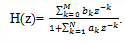Test: Filter Coefficients Quantization - Question 2

### If ak is the filter coefficient and a ̅k represents the quantized coefficient with Δak as the quantization error, then which of the following equation is true?

Detailed Solution for Test: Filter Coefficients Quantization - Question 2

Explanation: The quantized coefficient a ̅k can be related to the un-quantized coefficient ak by the relation
a ̅k = ak + Δak
where Δak represents the quantization error.

Test: Filter Coefficients Quantization - Question 3

### Which of the following is the equivalent representation of the denominator of the system function of a general IIR filter?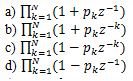Detailed Solution for Test: Filter Coefficients Quantization - Question 3

Explanation: We know that the system function of a general IIR filter is given by the equation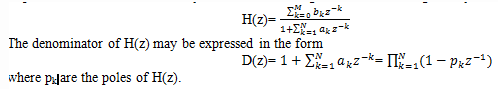Test: Filter Coefficients Quantization - Question 4

If pk is the set of poles of H(z), then what is Δpk that is the error resulting from the quantization of filter coefficients?

Detailed Solution for Test: Filter Coefficients Quantization - Question 4

Explanation: We know that p ̅k = pk + Δpk, k=1,2…N and Δpk that is the error resulting from the quantization of filter coefficients, which is called as perturbation error.

Test: Filter Coefficients Quantization - Question 5

What is the expression for the perturbation error Δpi?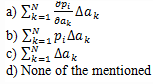Detailed Solution for Test: Filter Coefficients Quantization - Question 5

Explanation: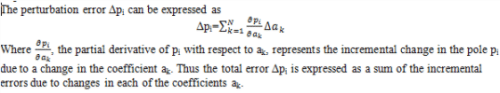Test: Filter Coefficients Quantization - Question 6

Which of the following is the expression for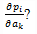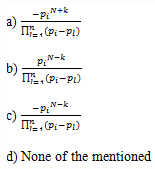Detailed Solution for Test: Filter Coefficients Quantization - Question 6

Explanation: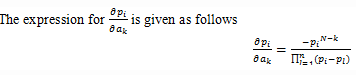Test: Filter Coefficients Quantization - Question 7

If the poles are tightly clustered as they are in a narrow band filter, the lengths of |pi-pl| are large for the poles in the vicinity of pi.

Detailed Solution for Test: Filter Coefficients Quantization - Question 7

Explanation: If the poles are tightly clustered as they are in a narrow band filter, the lengths of |pi-pl| are small for the poles in the vicinity of pi. These small lengths will contribute to large errors and hence a large perturbation error results.

Test: Filter Coefficients Quantization - Question 8

Which of the following operation has to be done on the lengths of |pi-pl| in order to reduce the perturbation errors?

Detailed Solution for Test: Filter Coefficients Quantization - Question 8

Explanation: The perturbation error can be minimized by maximizing the lengths of |pi-pl|. This can be accomplished by realizing the high order filter with either single pole or double pole filter sections.

Test: Filter Coefficients Quantization - Question 9

The sensitivity analysis made on the poles of a system results on which of the following of the IIR filters?

Detailed Solution for Test: Filter Coefficients Quantization - Question 9

Explanation: The sensitivity analysis made on the poles of a system results on the zeros of the IIR filters.

Test: Filter Coefficients Quantization - Question 10

Which of the following is the equivalent representation of the denominator of the system function of a general IIR filter?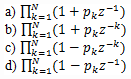Detailed Solution for Test: Filter Coefficients Quantization - Question 10

Explanation: We know that the system function of a general IIR filter is given by the equation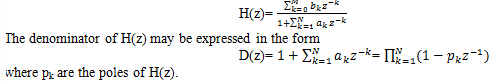## Signals and Systems

32 videos|76 docs|63 tests
 Use Code STAYHOME200 and get INR 200 additional OFF Use Coupon Code
Information about Test: Filter Coefficients Quantization Page
In this test you can find the Exam questions for Test: Filter Coefficients Quantization solved & explained in the simplest way possible. Besides giving Questions and answers for Test: Filter Coefficients Quantization, EduRev gives you an ample number of Online tests for practice

## Signals and Systems

32 videos|76 docs|63 tests